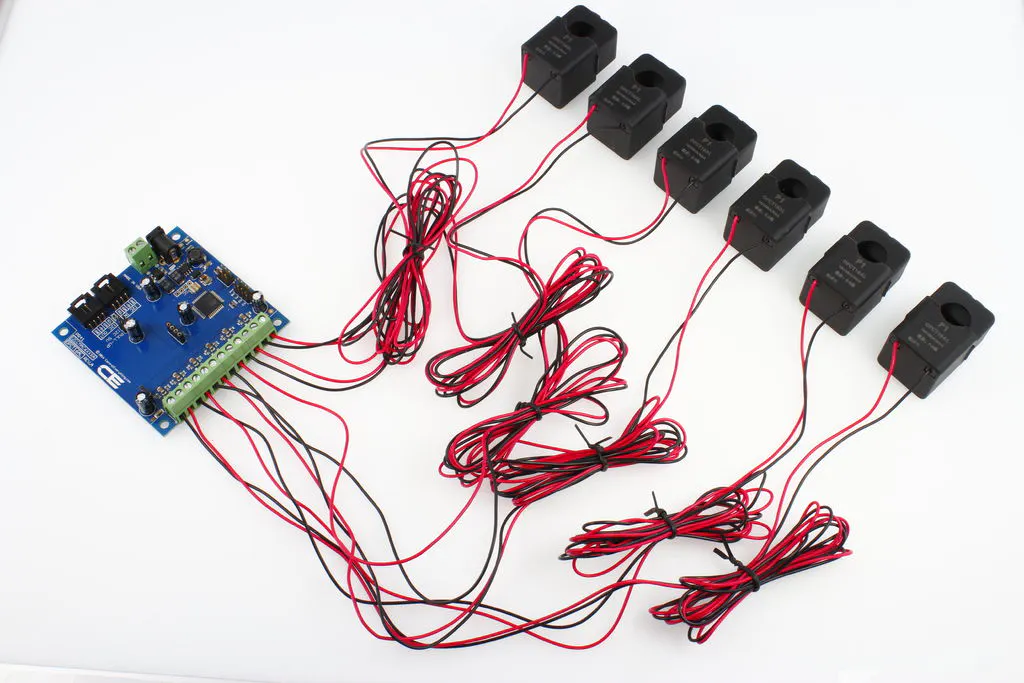Power monitoring, appliance detection using Arduino and save data into a Text file, Google Sheet for creating graphs and analysis.

IntermediateFull instructions provided2 hours12,951## Things used in this project

### Hardware components

 ControlEverything.com current mointoring controller
×1
 ControlEverything.com Arduino I2C hat
×1Arduino Nano R3
×1

### Software apps and online servicesArduino IDE## Schematics

### Current monitoring wiring diagram

this will help user in connecting sensor and in wiring

## Code

### Code snippet #1

Plain text
```//Include Wire I2C Library
#include  <wire.h>
double voltage = 121.7;   //////// voltage reading
double tkWh = 0;
double tWh = 0;
void setup()
{

Serial.begin(9600);  // Create Serial Object (9600 Baud)
Wire.begin();
}
void loop()
{

Wire.write(1);  // Command 1
Wire.write(1);  // Start Channel no
Wire.write(1);  // End Channel no
Wire.write(0);
Wire.write(0);
Wire.write((146 + 106 + 1 + 1 + 1 + 0 + 0) & 255); // CheckSum
Wire.endTransmission(); // Complete Transmission

MSB1= MSB1*65536;
MSB=MSB*256;
MSB1=MSB1+MSB+LSB;
double current = ((double)MSB1)/(double)1000;

Serial.print(current,3); //// current on ch 1
Serial.print(",");
double Power = voltage * current;  /// power on ch1
Serial.print(Power,3);
Serial.print(",");
double Wh = 0.000833 * Power;  /// Watt hour on ch1
Serial.print(Wh,4);
Serial.print(",");

tWh = tWh + Wh;   /// total watt hour used
Serial.print(tWh,4);
Serial.print(",");

tkWh = tWh/1000;  /// total kilo watt hour used
Serial.print(tkWh,4);
</p><p>
Serial.print("\n");

Wire.endTransmission(); // Complete Transmission

delay(3000);
}
```

### Code snippet #2

Plain text
```Serial.print(current,3); //// current on ch 1

Serial.print(",");
double Power = voltage * current;  /// power on ch1
Serial.print(Power,3);
Serial.print(",");
double Wh = 0.000833 * Power;  /// Watt hour on ch1
Serial.print(Wh,4);
Serial.print(",");

tWh = tWh + Wh;   /// total watt hour used
Serial.print(tWh,4);
Serial.print(",");

tkWh = tWh/1000;  /// total killo watt hour used
Serial.print(tkWh,4);
```